• 一、 命题逻辑基本概念 、 二、 等值演算 、 三、 主合取 ( 析取 ) 范式 、 四、 推理演算
文章目录一、 命题逻辑基本概念二、 等值演算三、 主合取 ( 析取 ) 范式四、 推理演算1、附加律2、化简律3、假言推理4、拒取式5、析取三段论6、假言三段论7、等价三段论8、构造性两难

参考博客 :

【数理逻辑】命题和联结词 ( 命题 | 命题符号化 | 真值联结词 | 否 | 合取 | 析取 | 非真值联结词 | 蕴涵 | 等价 )
【数理逻辑】命题逻辑 ( 命题与联结词回顾 | 命题公式 | 联结词优先级 | 真值表 可满足式 矛盾式 重言式 )
【数理逻辑】命题逻辑 ( 等值演算 | 幂等律 | 交换律 | 结合律 | 分配律 | 德摩根律 | 吸收率 | 零律 | 同一律 | 排中律 | 矛盾律 | 双重否定率 | 蕴涵等值式 … )
【数理逻辑】范式 ( 合取范式 | 析取范式 | 大项 | 小项 | 极大项 | 极小项 | 主合取范式 | 主析取范式 | 等值演算方法求主析/合取范式 | 真值表法求主析/合取范式 )
【数理逻辑】命题逻辑 ( 命题逻辑推理 | 推理的形式结构 | 推理定律 | 附加律 | 化简律 | 假言推理 | 拒取式 | 析取三段论 | 假言三段论 | 等价三段论 | 构造性两难 )
【数理逻辑】命题逻辑 ( 命题逻辑推理正确性判定 | 形式结构是永真式 - 等值演算 | 从前提推演结论 - 逻辑推理 )

一、 命题逻辑基本概念

命题逻辑基本概念

命题逻辑联结词
真值表
命题逻辑类型 : 可满足式 , 永真式 , 永假式 ;

1 . 命题公式 组成 :
① 单个 命题变元 / 命题常元 是命题公式 ;
② 如果 $A$ 是命题公式 , 则 $(\lnot A)$ 也是命题公式 ;
③ 如果 $A,B$ 是命题公式 , 则 $(A \land B) , (A \lor B), (A \to B), (A \leftrightarrow B)$  也是命题公式 ;
④ 有限次 应用 ① ② ③ 形成的符号串 是命题公式 ; ( 无限次不行 )

2 . 联结词 :
原子命题 : $p , q , r$ 表示 原子命题 , 又称为 简单命题 ;

真 : $1$ 表示 命题真值 为真 ;
假 : $0$ 表示 命题真值 为假 ;

联结词 : 上一篇博客 【数理逻辑】谓词逻辑 ( 个体词 | 个体域 | 谓词 | 全称量词 | 存在量词 | 谓词公式 | 习题 ) 三. 联结词  章节讲解了联结词 ;

否定联结词 : $\lnot$
合取联结词 : $\land$ , $p \land q$ , $pq$ 同真, 结果才为真 , 其余情况为假 ;
析取联结词 : $\lor$ , $p \lor q$ , $pq$ 同假, 结果才为假 , 其余情况为真 ;
蕴涵联结词 : $\to$ ,  $p \to q$ , $p$ 真 $q$ 假, 结果才为假 , 其余情况为真 ;
等价联结词 : $\leftrightarrow$ ,  $p \leftrightarrow q$ , $pq$ 真值相同时为真 , 表示等价成立 , 	$pq$ 真值相反时为假 , 等价不成立 ;

联结词优先级 :
“$\lnot$” 大于 “$\land , \lor$” 大于 “$\to, \leftrightarrow$”
$\land , \lor$ 优先级相同 ;
$\to, \leftrightarrow$ 优先级相同 ;

3 . 命题逻辑类型 :
可满足式 : 真值表中 , 至少有一个结果为真 , 可以都为真 ;
矛盾式 ( 永假式 ) : 所有的真值都为假 ;
可满足式 与 矛盾式 , 是 二选一 的 , 复合命题 要么是 可满足式 , 要么是 矛盾式 ;
重言式 ( 永真式 ) 是可满足式的一种 ;

4 . 简单命题形式化 :
参考 : 复合命题 与 命题符号化
定义命题 : 使用 $p,q$ 代表真假必居其一的陈述句 ;
使用联结词 : 然后使用联结词联结这些 $p,q$ 命题 ;

参考博客 :

【数理逻辑】命题和联结词 ( 命题 | 命题符号化 | 真值联结词 | 否 | 合取 | 析取 | 非真值联结词 | 蕴涵 | 等价 )
【数理逻辑】命题逻辑 ( 命题与联结词回顾 | 命题公式 | 联结词优先级 | 真值表 可满足式 矛盾式 重言式 )

二、 等值演算

等值式概念 : $A , B$ 是两个命题公式 , 如果 $A \leftrightarrow B$ 是永真式 , 那么 $A,B$ 两个命题公式是等值的 , 记做 $A \Leftrightarrow B$ ;
等值演算置换规则  : $A$ 和 $B$ 两个命题公式 , 可以 互相代替 , 凡是出现 $A$ 的地方都可以替换成 $B$ , 凡是出现 $B$ 的地方都可以替换成 $A$ ;

基本运算规律 :

1. 幂等律  : $A \Leftrightarrow A \lor A$ , $A \Leftrightarrow A \land A$
2. 交换律 : $A \lor B \Leftrightarrow B \lor A$ , $A \land B \Leftrightarrow B \land A$
3. 结合律 : $(A \lor B ) \lor C \Leftrightarrow A \lor (B \lor C)$ , $(A \land B ) \land C \Leftrightarrow A \land (B \land C)$
4. 分配律 : $A \lor (B \land C) \Leftrightarrow ( A \lor B ) \land ( A \lor C )$ , $A \land (B \lor C) \Leftrightarrow ( A \land B ) \lor ( A \land C )$

新运算规律 :

5. 德摩根律 : $\lnot ( A \lor B ) \Leftrightarrow \lnot A \land \lnot B$ , $\lnot ( A \land B ) \Leftrightarrow \lnot A \lor \lnot B$

有了 与 ( $\land$ ) 非 ( $\lnot$ ) , 就可以表示 或 ( $\lor$ )
有了 或 ( $\lor$ ) 非 ( $\lnot$ )  , 就可以表示 与 ( $\land$ )

6. 吸收率 :

前者将后者吸收了 : $A \lor ( A \land B ) \Leftrightarrow A$
后者将前者吸收了 :  $A \land ( A \lor B ) \Leftrightarrow A$ ;

$0 , 1$ 相关的运算律 :

7. 零律 : $A \lor 1 \Leftrightarrow 1$ , $A \land 0 \Leftrightarrow 0$

$1$ 是或运算的 零元 , $0$ 是与运算的 零元 ;
与 零元 进行运算结果是 零元 ;

8. 同一律 : $A \lor 0 \Leftrightarrow A$ , $A \land 1 \Leftrightarrow A$

$0$ 是或运算的 单位元 , $1$ 是 与运算的 单位元
与 单位元 进行运算结果是其 本身

9. 排中律 : $A \lor \lnot A \Leftrightarrow 1$
10. 矛盾律 : $A \land \lnot A \Leftrightarrow 0$

对偶原理适用于上述运算律 , 将两边的 $\land , \lor$ 互换 , 同时 $0 ,1$ 互换 , 等价仍然成立 ;

等价蕴含运算规律 :

11. 双重否定率 : $\lnot \lnot A \Leftrightarrow A$
12. 蕴涵等值式 : $A \to B \Leftrightarrow \lnot A \lor B$

替换蕴含联结词 : 蕴含联结词 $\to$ 不是必要的 , 使用 $\lnot , \lor$ 两个联结词可以替换 蕴含联结词 ;

13. 等价等值式 : $A \leftrightarrow B \Leftrightarrow ( A \to B ) \lor ( B \to A )$

双箭头 ( 等价联结词 ) 可以理解成重分必要条件
$A \to B$ ( 蕴含联结词 ) 理解成 $A$ 是 $B$ 的充分条件 , $B$ 是 $A$ 的必要条件
$B \to A$ ( 蕴含联结词 ) 理解成 $B$ 是 $A$ 的充分条件 , $A$ 是 $B$ 的必要条件
替换等价联结词 : 等价联结词 $\leftrightarrow$ 不是必要的 , 使用 $\to , \lor$ 两个联结词可以替换 等价联结词 ;

14. 等价否定等值式 : $A \leftrightarrow B \Leftrightarrow \lnot A \leftrightarrow \lnot B$
15. 假言易位 ( 逆否命题 ) : $A \to B \Leftrightarrow \lnot B \to \lnot A$

$A$ 称为 前件 , $B$ 称为 后件 ( 结论 ) ;

16. 归谬论 ( 反证法 ) : $( A \to B ) \land ( A \to \lnot B ) \Leftrightarrow \lnot A$

这是反证法的原理 , 由 $A$ 推导出 $B$ 和 $\lnot B$ , $B$ 和 $\lnot B$ 是矛盾的 , 则 $A$ 是错的 , $\lnot A$ 是对的 ;

参考博客 : 【数理逻辑】命题逻辑 ( 等值演算 | 幂等律 | 交换律 | 结合律 | 分配律 | 德摩根律 | 吸收率 | 零律 | 同一律 | 排中律 | 矛盾律 | 双重否定率 | 蕴涵等值式 … )

三、 主合取 ( 析取 ) 范式

1 . 极小项
极小项 : 极小项 是 一种 简单合取式 ;

1.前提 ( 简单合取式 ) : 含有 $n$ 个 命题变项 的 简单合取式 ;
2.命题变项出现次数 : 每个命题变项 均 以 文字 的 形式 在其中出现 , 且 仅出现 一次 ;
3.命题变项出现位置 : 第 $i$ ( $1 \leq i \leq n$ ) 个文字出现在 左起 第 $i$ 个位置 ;

$n$ 是指命题变项个数 ;

4.极小项总结 : 满足上述三个条件的 简单合取式 , 称为 极小项 ;
5.$m_i$ 与 $M_i$ 之间的关系 : ① $\lnot m_i \iff M_i$ ② $\lnot M_i \iff m_i$

每个命题 按照指定顺序 , 且 只出现一次 的 简单合取式 , 称为极小项 ;
极小项列出的是成真赋值 , 因为合取式只有一种情况成真 , 那就是全真 ;

2 . 极大项
关于 极大项 的 说明 :

1.极大项个数 :  $n$ 个 命题变元 会 产生 $2^n$ 个 极大项 ;
2.互不等值 : $2^n$ 个极大项 均 互不等值 ;
3.极大项 : $m_i$ 表示 第 $i$ 个极大项 , 其中 $i$ 是该极大项 成假赋值 的 十进制表示 ;
4.极大项名称 : 第 $i$ 个极大项 , 称为 $M_i$ ;
5.$m_i$ 与 $M_i$ 之间的关系 : ① $\lnot m_i \iff M_i$ ② $\lnot M_i \iff m_i$

每个命题 按照指定顺序 , 且 只出现一次 的 简单析取式 , 称为极小项 ;
极大项列出的是成假赋值 , 因为析取式只有一种情况成假 , 那就是全假 ;

3 . 主合取 ( 析取 ) 范式
① 列出要求 主合取 ( 析取 ) 范式 的真值表 ;
$p , q , r$ 三个命题真值从 $0,0,0$ 到 $1,1,1$ , 有 $2^3 = 8$ 列 , 每一列分别对应 $m_0 \sim m_8$ 极小项 , $M_0 \sim M_8$ 极大项 ;

② 主析取范式 ( 取极小项 ) : 真值表中的真值为 $1$ 的列 取 极小项 ; 极小项 成真赋值 ; 根据极小项下标与成真赋值可以列出极小项的命题公式 ;

③ 主合取范式 ( 取极大项 ) : 真值表中的真值为 $0$ 的列 取 极大项 ; 极大项 成假赋值 ; 根据极大项下标与成假赋值可以列出极大项的命题公式

4 . 总结 :
极小项 : 合取式 , 成真赋值 , 计算时取真值表 真 列 ;
极大项 : 析取式 , 成假赋值 , 计算时取真值表 假 列 ;

参考博客 : 【数理逻辑】范式 ( 合取范式 | 析取范式 | 大项 | 小项 | 极大项 | 极小项 | 主合取范式 | 主析取范式 | 等值演算方法求主析/合取范式 | 真值表法求主析/合取范式 )

四、 推理演算

推理的形式结构
前提 : $A_1 , A_2 , \cdots , A_k$
结论 : $B$
推理的形式结构为 : $(A_1 \land A_2 \land \cdots \land A_k) \to B$

推理定律 : $A,B$ 是两个命题 , 如果 $A \to B$ 是永真式 , 那么 $A \Rightarrow B$ ;

1、附加律

附加律 : $A \Rightarrow (A \lor B)$
根据 推理定律 , $A \to (A \lor B)$ 蕴含式 是 永真式 ;
前提 :  $A$
结论 : $A \lor B$

$A$ 是对的 , 那么 $A \lor B$ 也是对的 , 后者是在前者基础上附加了一个 $B$ ;

2、化简律

化简律 : $( A \land B ) \Rightarrow A$ , $( A \land B ) \Rightarrow B$
根据 推理定律 , $( A \land B ) \to A$ ,  $( A \land B ) \to B$ 蕴含式 是 永真式 ;
前提 :  $A \land B$
结论 : $A$ 或 $B$

$A \land B$ 是对的 , 那么 $A$ 或 $B$ 也是对的 , 后者是在前者基础上进行了化简 ;

3、假言推理

假言推理 : $( A \to B ) \land A \Rightarrow B$
根据 推理定律 , $( A \to B ) \land A \to B$ 蕴含式 是 永真式 ;
前提 :  $A \to B$ , $A$
结论 : $B$

这是个典型的小三段论 ;

4、拒取式

拒取式: $( A \to B ) \land \lnot B \Rightarrow \lnot A$
根据 推理定律 , $( A \to B ) \land \lnot B \to \lnot A$ 蕴含式 是 永真式 ;
前提 :  $A \to B$ , $\lnot B$
结论 : $\lnot A$

可以理解为是反证法 ;

5、析取三段论

析取三段论 : $( A \lor B ) \land \lnot A \Rightarrow B$ , $( A \lor B ) \land \lnot B \Rightarrow A$
根据 推理定律 , $( A \lor B ) \land \lnot A \to B$ , $( A \lor B ) \land \lnot B \to A$ 蕴含式 是 永真式 ;
前提 :  $A \lor B$ , $\lnot A$
结论 : $B$

$(A \lor B)$ 是正确的 , 其中 $A$ 是错误的 , 那么 $B$ 肯定是正确的 ;
$(A \lor B)$ 是正确的 , 其中 $B$ 是错误的 , 那么 $A$ 肯定是正确的 ;
警察破案常用推理方式 , 逐一排除嫌疑人 ;

6、假言三段论

假言三段论 : $( A \to B ) \land ( B \to C ) \Rightarrow ( A \to C )$
根据 推理定律 , $( A \to B ) \land ( B \to C ) \to ( A \to C )$ 蕴含式 是 永真式 ;
前提 :  $A \to B$ , $B \to C$
结论 : $A \to C$

7、等价三段论

等价三段论: $( A \leftrightarrow B ) \land ( B \leftrightarrow C ) \Rightarrow ( A \leftrightarrow C )$
根据 推理定律 , $( ( A \leftrightarrow B ) \land ( B \leftrightarrow C ) ) \to ( A \leftrightarrow C )$ 蕴含式 是 永真式 ;
前提 :  $A \leftrightarrow B$ , $B \leftrightarrow C$
结论 : $A \leftrightarrow C$

8、构造性两难

等价三段论: $( A \to B ) \land ( C \to D ) \land ( A \lor C ) \Rightarrow ( B \lor D )$
根据 推理定律 , $( ( A \to B ) \land ( C \to D ) \land ( A \lor C ) ) \to ( ( B \lor D ) )$ 蕴含式 是 永真式 ;
前提 :  $A \to B$ , $C \to D$ ,  $A \lor C$
结论 : $B \lor D$

理解方式 :
$A$ 是发展经济 , $B$ 是污染
$C$ 是不发展经济 , $D$ 是贫穷
$A \lor B$ 要么发展经济 , 要么不发展经济
结果是 $B \lor D$ , 要么产生污染 , 要么忍受贫穷

参考博客 : 【数理逻辑】命题逻辑 ( 命题逻辑推理 | 推理的形式结构 | 推理定律 | 附加律 | 化简律 | 假言推理 | 拒取式 | 析取三段论 | 假言三段论 | 等价三段论 | 构造性两难 )


展开全文主合取范式 主析取范式
• 文章目录主要内容一 等值式等值式基本等值式等值演算与置换规则等值演算的应用举例二 析取范式与合取范式范式的性质极小项与极大项实例主析取范式与主合取范式求公式主范式的步骤实例主范式的应用三 联结词的完备集...
离散数学与组合数学汇总
文章目录主要内容一 等值式1 等值式2 等值式例题3 基本等值式4  等值演算与置换规则5 等值演算的应用举例二 析取范式与合取范式1 范式的性质2 极小项与极大项3 实例4 主析取范式与主合取范式5 求公式主范式的步骤6 实例7 主范式的应用1.求公式的成真成假赋值2.  判断公式的类型3.  判断两个公式是否等值4. 解实际问题三 联结词的完备集真值函数联结词完备集复合联结词四 可满足性问题与消解法消解规则消解序列与合取范式的否证消解算法消解算法例题
主要内容

等值式与基本的等值式
等值演算与置换规则
析取范式与合取范式，主析取范式与主合取范式
联结词完备集
可满足性问题与消解法

一 等值式
1 等值式
定义2.1 若等价式A↔B是重言式，则称A与B等值，记作A<=>B，并称A<=>B是等值式

几点说明：

定义中，A, B, <=>均为元语言符号
A或B中可能有哑元出现.
例如    (p→q)<=> (( ¬p∨q)∨(¬r∧r))     r为左边公式的哑元.
用真值表可检查两个公式是否等值
请验证：
p→(q→r) <=> (p∧q) →r
p→(q→r) 不与 (p→q) →r 等值

2 等值式例题
例1 判断下列各组公式是否等值:
(1)  p→(q→r) 与 (p∧q) →r结论:  p→(q→r) <=> (p∧q) →r
(2)   p→(q→r) 与 (p→q) →r结论：p→(q→r) 与 (p→q) →r  不等值
3 基本等值式

双重否定律  			¬¬A<=>A
幂等律        A∨A<=>A,  A∧A<=>A
交换律        A∨B<=>B∨A,  A∧B<=>B∧A
结合律        (A∨B)∨C<=>A∨(B∨C),  (A∧B)∧C<=>A∧(B∧C)
分配律        A∨(B∧C)<=>(A∨B)∧(A∨C),
A∧(B∨C)<=>(A∧B)∨(A∧C)
德摩根律    ¬(A∨B)<=>¬A∧¬B
¬(A∧B)<=>¬A∨¬B
吸收律        A∨(A∧B)<=>A,  A∧(A∨B)<=>A
零律                       A∨1<=>1,   A∧0<=>0
同一律                   A∨0<=>A.  A∧1<=>A
排中律                   A∨¬A<=>1
矛盾律                   A∧¬A<=>0
蕴涵等值式           A→B<=>¬A∨B
等价等值式           A↔B<=>(A→B)∧(B→A)
假言易位               A→B<=>¬B→¬A
等价否定等值式   A↔B<=>¬A↔¬B
归谬论                  (A↔B)∧(A→¬B) <=>¬A

特别提示：必须牢记这16组等值式，这是继续学习的基础
4  等值演算与置换规则

等值演算——由已知的等值式推演出新的等值式的过程
等值演算的基础：
(1) 等值关系的性质：自反性、对称性、传递性
(2) 基本的等值式
(3) 置换规则（见3）
置换规则
设  Φ(A) 是含公式 A 的命题公式，Φ(B) 是用公式 B 置换
Φ(A) 中所有的 A 后得到的命题公式
若 B<=>A，则Φ(B)<=>Φ(A)

5 等值演算的应用举例
证明两个公式等值

例2 证明 p→(q→r) <=> (p∧q)→r

证      p→(q→r)
<=> ¬p∨（¬q∨r)     （蕴涵等值式，置换规则）
<=> (¬p∨¬q)∨r     （结合律，置换规则）
<=> ¬(p∧q)∨r      （德摩根律，置换规则）
<=> (p∧q)→r       （蕴涵等值式，置换规则）
今后在注明中省去置换规则
注意：用等值演算不能直接证明两个公式不等值

证明两个公式不等值

例3  证明 p→(q→r) 与 (p∧q)→r 不等值

证
方法一 真值表法, 见例1(2)
方法二 观察法. 观察到000, 010是左边的成真赋值，是右边的成假赋值
方法三 先用等值演算化简公式，然后再观察
p→(q→r) <=>¬p∨¬q∨r
(p→q)→r <=>¬(¬p∨q)∨r<=>(p∧¬q)∨r
更容易看出前面的两个赋值分别是左边的成真赋
值和右边的成假赋值
判断公式类型: A为矛盾式当且仅当A <=> 0
A为重言式当且仅当A <=>1

例4  用等值演算法判断下列公式的类型
(1)  q∧¬(p→q)
(2)  (p→q) ↔(¬q→¬p)
(3)  ((p∧q)∨(p∧¬q))∧r)

解  (1)   q∧¬(p→q)
<=> q∧¬(¬p∨q)   （蕴涵等值式）
<=> q∧(p∧¬q)     （德摩根律）
<=> p∧(q∧¬q)     （交换律，结合律）
<=> p∧0               （矛盾律）
<=> 0                   （零律）
矛盾式
(2)   (p→q) ↔(¬q→¬p)
<=> (¬p∨q)↔(q∨¬p)    （蕴涵等值式）
<=> (¬p∨q)↔(¬p∨q)    （交换律）
<=> 1
重言式
(3) ((p∧q)∨(p∧¬q))∧r)
<=>  (p∧(q∨¬q))∧r       （分配律）
<=>  p∧1∧r                   （排中律）
<=>  p∧r                       （同一律）
可满足式，101和111是成真赋值，000和010等是成假赋值.

二 析取范式与合取范式
基本概念
(1) 文字——命题变项及其否定的总称
(2) 简单析取式——有限个文字构成的析取式
p,  ¬q,  p∨¬q,  p∨q∨r, …
(3) 简单合取式——有限个文字构成的合取式
p,  ¬q,  p∧¬q,  p∧q∧r, …
(4) 析取范式——由有限个简单合取式组成的析取式
p, ¬p∧q,  p∨¬q,  (p∧¬q)∨(¬p∧q∧¬r)∨(q∧r)
(5) 合取范式——由有限个简单析取式组成的合取式
p,   p∨¬q,  ¬p∧q,  (p∨q∧¬p)∧(p∨¬q∨¬r)
(6) 范式——析取范式与合取范式的总称
说明：

单个文字既是简单析取式，又是简单合取式
形如 p∧¬q∧r,  ¬p∨q∨¬r 的公式既是析取范式，又是合取范式

1 范式的性质
定理2.1 (1) 一个简单析取式是重言式当且仅当它同时含有某个命题变项和它的否定式.
(2) 一个简单合取式是矛盾式当且仅当它同时含有某个命题变项和它的否定式.
定理2.2  (1) 一个析取范式是矛盾式当且仅当它每个简单合取式都是矛盾式.
(2) 一个合取范式是重言式当且仅当它的每个简单析取式都是重言式.
定理2.3（范式存在定理）
任何命题公式都存在与之等值的析取范式与合取范式
公式A的析取(合取)范式-与A等值的析取(合取)范式
求公式A的范式的步骤
(1) 消去A中的→, ↔（若存在）
A→B<=>¬A∨B
A↔B<=>(¬A∨B)∧(A∨¬B)
(2) 否定联结词¬的内移或消去
¬ ¬A<=> A
¬(A∨B)<=> ¬A∧¬B
¬(A∧B)<=> ¬A∨¬B
(3) 使用分配律
A∨(B∧C)<=> (A∨B)∧(A∨C)             求合取范式
A∧(B∨C)<=>  (A∧B)∨(A∧C)            求析取范式
公式范式的不足——不惟一

例5  求下列公式的析取范式与合取范式
(1) (p→¬q)∨¬r
(2) (p→¬q)→r
解   (1)  (p→¬q)∨¬r
<=>   (¬p∨¬q)∨¬r     （消去→）
<=>   ¬p∨¬q∨¬r       （结合律）
最后结果既是析取范式(由3个简单合取式组成的析取式)，又
是合取范式(由一个简单析取式组成的合取式)
(2)  (p→¬q)→r
<=>  (¬p∨¬q)→r     （消去第一个→）
<=> ¬(¬p∨¬q)∨r    （消去第二个→）
<=>  (p∧q)∨r            （否定号内移——德摩根律)   析取范式
<=>  (p∨r)∧(q∨r)    （∨对∧分配律）   合取范式

2 极小项与极大项
定义2.4 在含有n个命题变项的简单合取式（简单析取式）
中，若每个命题变项均以文字的形式在其中出现且仅出现
一次，而且第i个文字出现在左起第i位上（1<=i<=n），称这
样的简单合取式（简单析取式）为极小项（极大项）.

几点说明：
n个命题变项有2n个极小项和2n个极大项
2n个极小项（极大项）均互不等值
用mi表示第i个极小项，其中i是该极小项成真赋值的十进制表示. 用Mi表示第i个极大项，其中i是该极大项成假赋值的十进制表示. mi（Mi）称为极小项（极大项）的名称.

3 实例
由两个命题变项 p, q 形成的极小项与极大项由三个命题变项 p, q, r 形成的极小项与极大项.mi与Mi的关系： ¬mi <=> Mi,    ¬Mi <=> mi
4 主析取范式与主合取范式
主析取范式——由极小项构成的析取范式
主合取范式——由极大项构成的合取范式

例如，n=3, 命题变项为 p, q, r 时，
(¬p∧¬q∧r)∨(¬p∧q∧r) <=> m1∨m3 ——主析取范式
(p∨q∨¬r)∧(¬p∨¬q∨¬r) <=> M1∨M7——主合取范式
公式A的主析取(合取)范式——与A 等值的主析取(合取)范式

定理2.5  (主范式的存在惟一定理)
任何命题公式都存在与之等值的主析取范式和主合取范式,
并且是惟一的
5 求公式主范式的步骤
求公式主析取范式的步骤:
设公式A含命题变项p1,p2,…,pn
(1) 求A的析取范式A’=B1∨ B2∨ … ∨ Bs , 其中Bj是简单合取
式 j=1,2, … ,s
(2) 若某个Bj既不含pi, 又不含¬pi, 则将Bj展开成
Bj <=> Bj∧(pi∨¬pi) <=> (Bj∧pi)∨(Bj∧¬ pi)
重复这个过程, 直到所有简单合取式都是长度为n的极
小项为止
(3) 消去重复出现的极小项, 即用mi代替mi∧mi
(4) 将极小项按下标从小到大排列
6 实例
例6  (1) 求公式 A=(p→¬q)→r的主析取范式和主合取范式7 主范式的应用
1.求公式的成真成假赋值
设公式A含n个命题变项, A的主析取范式有s个极小项, 则A
有s个成真赋值, 它们是极小项下标的二进制表示, 其余2n-s
个赋值都是成假赋值

例如   (p→¬q)→r <=>  m1∨m3∨m5∨ m6∨m7
成真赋值为 001, 011, 101, 110, 111，
成假赋值为 000, 010, 100.
类似地，由主合取范式也立即求出成假赋值和成真赋值.
2.  判断公式的类型
设A含n个命题变项.
A为重言式<=> A的主析取范式含全部2n个极小项
<=> A的主合取范式不含任何极大项, 记为1.
A为矛盾式 <=> A的主合析取范式含全部2n个极大项
<=> A的主析取范式不含任何极小项, 记为0.
A为非重言式的可满足式
<=>A的主析取范式中至少含一个、但不是全
部极小项
<=>A的主合取范式中至少含一个、但不是全
部极大项.

例7 用主析取范式判断公式的类型:3.  判断两个公式是否等值
例8  用主析取范式判以下每一组公式是否等值显见，⑴中的两公式等值，而⑵的不等值.
4. 解实际问题

例9 某单位要从A,B,C三人中选派若干人出国考察, 需满足下
述条件:
(1) 若A去, 则C必须去;
(2) 若B去, 则C不能去;
(3) A和B必须去一人且只能去一人.
问有几种可能的选派方案?
解  记 p:派A去,  q:派B去, r:派C去
(1)  p→ r,   (2) q→ ¬r,   (3) (p∧¬q)∨(¬p∧q)
求下式的成真赋值
A=(p→r)∧(q→¬r)∧((p∧¬q)∨(¬p∧q))
求A的主析取范式成真赋值:101,010
结论: 方案1  派A与C去,  方案2  派B去
由主析取范式确定主合取范式
例10 设A有3个命题变项, 且已知A= m1∨m3∨m7, 求A的主合取
范式.
解   A的成真赋值是1,3,7的二进制表示, 成假赋值是在主析取
范式中没有出现的极小项的下角标0,2,4,5,6的二进制表示, 它
们恰好是A的主合取范式的极大项的下角标, 故
A  <=>  M0∧M2∧M4∧M5∧M6
由主合取范式确定主析取范式
用真值表确定主范式
三 联结词的完备集
真值函数
定义2.6  称F:{0,1}^n→ {0,1} 为n元真值函数.
{0,1} ^n   ={00…0, 00…1, …, 11…1}，包含
2^（2 ^n）次个长为n的0,1符号串.
共有 2的（2的n次）个n元真值函数.
1元真值函数2元真值函数任何一个含n个命题变项的命题公式A都对应惟一的一个n元
真值函数 F , F 恰好为A的真值表.
等值的公式对应的真值函数相同.
例如：p→ q, ¬p∨q  都对应F13（2）
联结词完备集
定义2.7  设S是一个联结词集合，如果任何n(n>=1) 元真值函
数都可以由仅含S中的联结词构成的公式表示，则称S是联结
词完备集
若S是联结词完备集, 则任何命题公式都可由S中的联结词表示
定理2.6  S = { ¬,∧ ,∨}是联结词完备集

证明  由范式存在定理可证
推论 以下都是联结词完备集
(1) S1 = {¬,∧ ,∨,→}          (2) S2 = {¬,∧ ,∨,→, ↔}
(3) S3 = {¬,∧}                    (4) S4 = {¬,∨}
(5) S5 = {¬, →}
证明
(1),(2) 在联结词完备集中加入新的联结词后仍为完备集
(3)  A∨B <=> ¬(¬A∧¬B)
(4)  A∧B <=> ¬(¬A∨¬B)
(5)  A→B<=>¬A∨B
{∧,∨,→,↔}不是联结词完备集, 0不能用它表示
它的子集{∧},{∨},{→},{↔},{∧,∨},{∧,∨,→}等都不是

复合联结词
定义2.8  设 p, q 为两个命题,¬(p∧q)称作p与q的与非式, 记作
p↑q, 即 p↑q <=> ¬(p∧q), ↑称为与非联结词
¬(p∨q) 称作 p 与 q 的或非式, 记作 p↓q, 即 p↓q  <=>¬(p∨q), ↓
称为或非联结词
定理2.7  {↑}与{↓}为联结词完备集.

证明  {¬, ∧, ∨}为完备集
¬p <=> ¬p∧¬p <=>  ¬(p∨p)<=>  p↓p
p∧q <=> ¬(¬p∨¬q) <=>  ¬p↓¬q <=>  (p↓p)↓(q↓q)
p∨q <=>¬¬(p∨q) <=>  ¬(p↓q) <=>  (p↓q)↓(p↓q)
得证{↓}为联结词完备集.  对{↑}类似可证

四 可满足性问题与消解法
不含任何文字的简单析取式称作空简单析取式,记作λ.

规定λ是不可满足的.
约定:简单析取式不同时含某个命题变项和它的否定
S:合取范式,  C:简单析取式,   l:文字,   α:赋值,    带下角标或 ’
文字l的补lc:若l=p,则lc=¬p;若l=¬p,则lc=p.
S≈S’:S是可满足的当且仅当S’ 是可满足的

消解规则
定义2.9 设C1=l∨C1’, C2=lc∨C2’, C1’和C2’不含l和lc, 称C1’∨C2’为
C1和C2(以l和lc为消解文字)的消解式或消解结果, 记作
Res(C1,C2)
例如, Res(¬p∨q∨r, p∨q∨¬s)= q∨r∨¬s消解序列与合取范式的否证消解算法消解算法例题
例12 用消解算法判断下述公式是否是可满足的:展开全文析取范式与合取范式
• 一阶逻辑等值演算与推理一阶逻辑等值一阶逻辑等值演算与推理演算与推理
• ## 命题逻辑等值演算

千次阅读 2018-07-12 22:26:37
命题逻辑等值演算   一、等值式 定义1 设A，B是两个命题公式，若A，B构成的等价式为重言式，则称A与B是等值的，记作。   16组常用的重要等值式模式： 1.双重否定律： 2.幂等律： 3.交换律： 4.结合律： ...
作者：离散梦

欢迎大家给出宝贵的建议！

命题逻辑等值演算

一、等值式

定义1

设A，B是两个命题公式，若A，B构成的等价式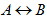为重言式，则称A与B是等值的，记作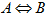。

16组常用的重要等值式模式：

1.双重否定律：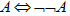2.幂等律：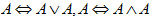3.交换律：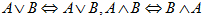4.结合律：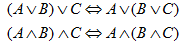5.分配率：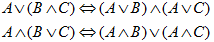6.德摩根律：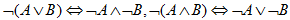7.吸收律：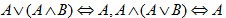8.零律：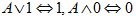9.同一律：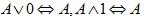10.排中律：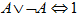11.矛盾律：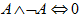12.蕴涵等值式：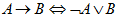13.等价等值式：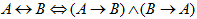14.假言易位：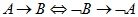15.等价否定等值式：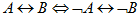16.归谬论：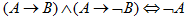由已知的等值式推演出另外一些等值式的过程称作等值演算。

二、析取范式与合取范式

定义2

命题变项及其否定统称作文字。仅由有限个文字构成的析取式称作简单析取式。仅由有限个文字构成的合取式称作简单合取式。

定义3

由有限个简单合取式的析取构成的命题公式称作析取范式。由有限个简单合取式的合取构成的命题公式称作合取范式。析取范式与合取范式统称作范式。

求给定公式范式的步骤为：

1.消去联结词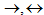。

2.用双重否定律消去双重否定符，用德摩根律内移否定符。

3.使用分配率：求析取范式时使用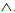对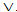的分配律，求合取范式时使用对的分配律。

定义4

在含有n个命题变项的简单合取式（简单析取式）中，若每个命题变项和它的否定式恰好出现一个且仅出现一次，而且命题变项或它的否定式按照下标从小到大或按照字典顺序排列，称这样的简单合取式（简单析取式）为极小项（极大项）。

定义5

所有简单合取式（简单析取式）都是极小项（极大项）的析取范式（合取范式）称为主析取范式（主合取范式）。

1.求公式的成真赋值与成假赋值。

如，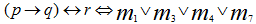，这里有3个命题变项，将主析取范式中各极小项的角标1,3,4,7写成长为3的二进制数，它们分别为001,011,100,111。这4个赋值即为该公式的成真赋值。而主析取范式中未出现的极小项m0,m2,m5,m6的角标的二进制表示000,010,101,110为该公式的成假赋值。

2.判断公式的类型

设公式A中含n个命题变项，容易看出：

（1）A为重言式当且仅当A的主析取范式含全部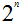个极小项。

（2）A为矛盾式当且仅当A的主析取范式不含任何极小项。此时，记A的主析取范式为0。

（3）A为可满足式当且仅当A的主析取范式中至少含有一个极小项。

三、联结词的完备集

定义6

称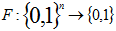为n元真值函数。

定义7

设S是一个联结词集合，如果任何n(n>1)元真值函数都可以由仅含S中的联结词构成的公式表示，则称S是联结词完备集。

定义8

设p，q两个命题，复合命题“p与q的否定式”称作p，q的与非式，记作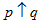，即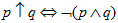。符号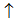称作与非联结词。复合命题“p或q的否定式”，称作p，q的或非式，记作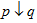。即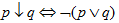。符号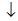称作或非联结词。

四、可满足性问题与消解法

称不含有任何文字的简单析取式为空简单析取式。
展开全文析取 合取
• 附上运行截图： 附上代码： #include <iostream> #include <cstdio> using namespace std; void printtitle(char a,char b,char c,int one,int two) ... case 0:printf("~%c",...
附上运行截图：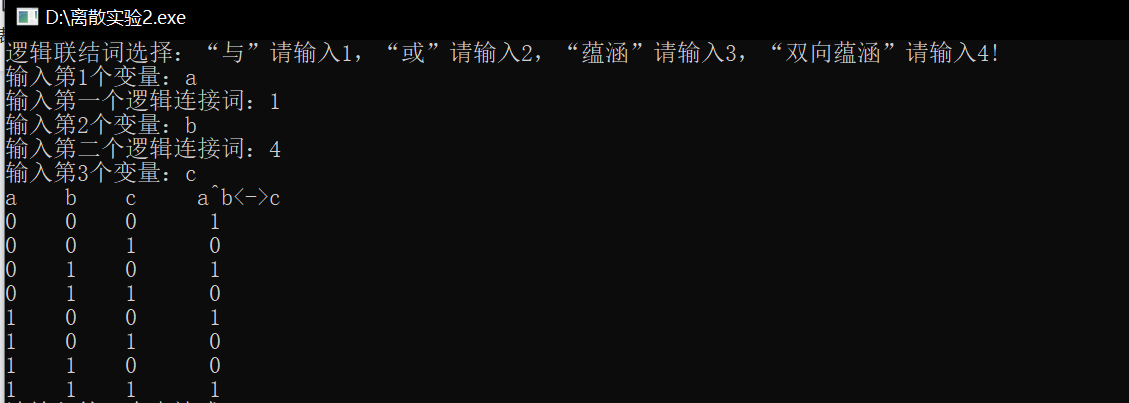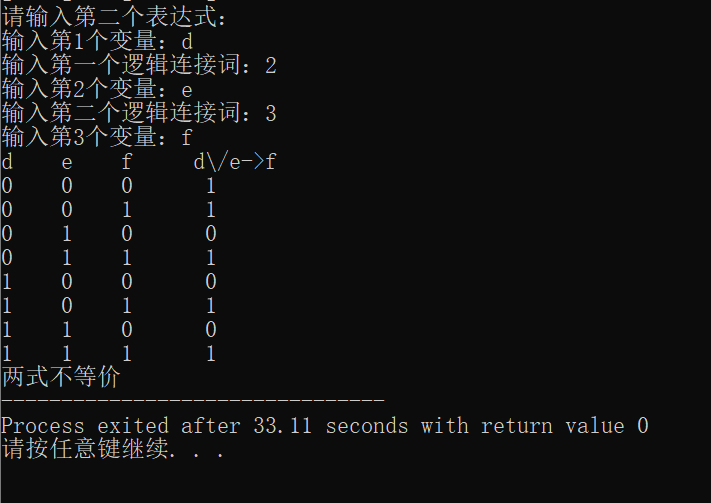附上代码：

#include <iostream>
#include <cstdio>
using namespace std;
void printtitle(char a,char b,char c,int one,int two)
{
printf("%c    %c    %c     %c",a,b,c,a);
switch(one)
{
case 0:printf("~%c",b);break;
case 1:printf("^%c",b);break;
case 2:printf("\\/%c",b);break;
case 3:printf("->%c",b);break;
case 4:printf("<->%c",b);break;
}
switch(two)
{
case 0:printf("~%c\n",c);break;
case 1:printf("^%c\n",c);break;
case 2:printf("\\/%c\n",c);break;
case 3:printf("->%c\n",c);break;
case 4:printf("<->%c\n",c);break;
}
}
int yunsuan(int p,int q,int connective)
{
if(connective==1)
{
return p*q;
}
else if(connective==2)
{
return ((p+q)/2+(p+q)%2);
}
else if(connective==3)
{
if(p==0)
return 1;
else
{
if(q==1)
return 1;
else
return 0;
}
}
else if(connective==4)
{
if(p==q)
return 1;
else
return 0;
}
}

int main()
{
char a,b,c,d,e,f;
int s,x;
int count1=0,count2=0;
int m,n,p,q;
cout<<"逻辑联结词选择：“与”请输入1，“或”请输入2，“蕴涵”请输入3，“双向蕴涵”请输入4!"<<endl;
cout<<"输入第1个变量：";         cin>>a;
cout<<"输入第一个逻辑连接词：";  cin>>m;
cout<<"输入第2个变量：";         cin>>b;
cout<<"输入第二个逻辑连接词：";  cin>>n;
cout<<"输入第3个变量：";         cin>>c;
printtitle(a,b,c,m,n);

int i,j,k;
for (i=0;i<2;i++)
{
for (j=0;j<2;j++)
{
for (k=0;k<2;k++)
{
printf("%d    %d    %d      ",i,j,k);
if(m<=n)
{
s[count1]=yunsuan(yunsuan(i,j,m),k,n);
count1++;
cout<<yunsuan(yunsuan(i,j,m),k,n)<<endl;
}

else
{
s[count1]=yunsuan(i,yunsuan(j,k,n),m);
count1++;
cout<<yunsuan(i,yunsuan(j,k,n),m)<<endl;
}

}
}
}

cout<<"请输入第二个表达式："<<endl;
cout<<"输入第1个变量：";         cin>>d;
cout<<"输入第一个逻辑连接词：";  cin>>p;
cout<<"输入第2个变量：";         cin>>e;
cout<<"输入第二个逻辑连接词：";  cin>>q;
cout<<"输入第3个变量：";         cin>>f;
printtitle(d,e,f,p,q);

for (i=0;i<2;i++)
{
for (j=0;j<2;j++)
{
for (k=0;k<2;k++)
{
printf("%d    %d    %d      ",i,j,k);
if(p<=q)
{
x[count2]=yunsuan(yunsuan(i,j,p),k,q);
count2++;
cout<<yunsuan(yunsuan(i,j,p),k,q)<<endl;
}

else
{
x[count2]=yunsuan(i,yunsuan(j,k,q),p);
count2++;
cout<<yunsuan(i,yunsuan(j,k,q),p)<<endl;
}

}
}
}

int flag=0;
for(int t=0;t<8;t++)
{
if(s[t]!=x[t])
{
flag=1;
break;
}
}
if(flag == 1)
cout<<"两式不等价";
else
cout<<"两式等价";
return 0;

}

下面总结一下上面的代码：

这次实验中我改编了实验一代码进行了两次输入和处理操作，然后将两次的处理结果用两个数组分别存储，然后在最后比较两个数组是否一样，若一样，则代表两式真值完全一样，输出“两式等价”。若数组不一样，则代表两式真值不一样，输出“两式不等价”。由于实验一代码可以对任意三种变量的所有情况进行处理，所以本次实验代码可以对任意两个关系式进行真值比较。

展开全文• 本文目录等值式基本等值式等值演算与置换规则等值演算置换规则等值演算的基础举个例子例1例2例33.1 q∧\wedge∧¬\neg¬(p->q)3.2 (p->q)<->(¬\neg¬q->p) 等值式 定义 ：若等价式A<->B是重言...
• 1.3 等值演算 定义：当且仅当A，B具有相同真值表时，A ↔\leftrightarrow↔ B 为永真式。此时称A与B是等值的，记作 ： A ⇔\Leftrightarrow⇔ B 注意： “⇔\Leftrightarrow⇔” 与 “=”不同，“A=B”表示...
• 第二章命题逻辑等值演算 2.1等值式 设公式A,B共同含有n个命题变项,A或B可能有哑元.若A与B有相同的真值表,则说明在所有2n个赋值下,A与B的真值都相同,因而等价式A<->B为重言式 定义2.1设A,B是两个命题...离散数学 数理逻辑
• 命题逻辑等值演算 等值式 暴力方法1：判断两个公式A与B是否等职的方法，最直接就是用真值表法判断$A\leftrightarrow B$是否为重言式。 16组常用的重要等值式模式： 双重否定律 A⇔¬¬AA \Leftrightarrow \neg \...离散数学
• Refer 五重循环遍历全部结果 5^5 5名跳水高手参加跳水决赛排名 默宇宇 2015-03-11 5位运动员参加了10米台跳水比赛，有人让他们预测比赛结果 A选手说：B第二，我第三； B选手说：我第二，E第...使用逻辑等值演算简化代码离散数学
• 一阶逻辑等值演算与推理 一阶逻辑中的基本等值式： 第一组：16组等值式给出的代换实例都是一阶逻辑的等值式。 第二组： 量词否等等值式 ¬∀xA(x)⇔∃x¬A(x)\neg \forall xA(x) \Leftrightarrow \exists x \neg A(x...离散数学 一阶逻辑 数理逻辑
• 求公式主析取范式的方法步骤:方法一：等值演算设公式A含命题变项p1,p2,…,pn(1) 求A的析取范式A=B1B2 … Bs , 其中Bj是简单合取式 j=1,2, … ,s(2) 若某个Bj既不含pi, 又不含Øpi, 则将Bj展开成 Bj ...
• 一、等值演算 、 二、等值式 、 三、基本等值式 、 四、基本运算 、 五、等值演算基本等值式 数理逻辑 德摩根律
• Chapter Two - 命题逻辑等值演算 1 - 要点 等值式：如果A↔B是重言式，那么称A与B等值的，记为A⇔B，并称A⇔B为等值式 基本等值式： （1）双重否定律 A⇔￢￢A （2）幂等律 A∨A⇔A，A∧A⇔A （3）交换律 A∨B⇔B∨A...数理逻辑
• ## 一阶逻辑等值演算

千次阅读 2015-04-22 22:11:47
设A，B是一阶逻辑中任意两个公式，若AB是永真式，则称A与B是等值的。记做AB，称AB是等值式。谓词逻辑中关于联结词的等值式与命题逻辑中相关等值式类似。下面主要讨论关于量词的等值式。基本等值式 第一组 代换实例 ...
• 一、 命题逻辑推理正确性判定 、 二、 形式结构是永真式 ( 等值演算 ) 、 三、 从前提推演结论 ( 逻辑推理 ) 、逻辑推理 离散数学
• 本资料为原书第2版PDF转为WORD形式而成，仅供学习参考！
•抽象代数
• 文章目录主要内容5.1 一阶逻辑等值式与置换规则5.2 置换规则、换名规则、代替规则1. 置换规则2. 换名规则3. 代替规则5.2 一阶逻辑前束范式5.3 一阶逻辑的推论理论 主要内容 一阶逻辑等值式与基本的等值式 置换规则...前束范式 自然推理系统
• 常用的等值式如下： 幂等律： 结合律： 交换律： 分配律： 双重否定句： 吸收率： 零率： 同一律： 补余律： 德摩根率： 等值演算 等值关系是一个等价关系，正是由于这种性质，使得我们可以从某个逻辑公式出发，...算法
• 情景： 记录数组中的最大值。 思路： 使用 last 来存放之前的最大值，使用 new 表示新的值，通过判断两者的大小来决定是否更新最大值 last。 最直接 // 如果 last 为空，或者 last 小于 new 的时候更新 ...
•数学
• 1. 等值式 定义2.1 设A,B是两个命题公式，若A，B构成的等价式A<->B为重言式，则称A与B是等值的，记作A<=>B. <=>不是连接符，它是用来说明A与B等值的一种记法，因而它是元语言符号。 本书给出16组...
• 设A, B是两个谓词公式, 如果AB是永真式, 则称A与B等值, 记作AB, 并称AB是等值式 基本等值式 第一组 命题逻辑中16组基本等值式的代换实例以及推理定律的代换实例 第二组 (1) 消去量词等值...
• 2.1 等值式 概念：若等价式AB是重言式，则称 A 与 B 等值, 记作 AB，并称AB是等值式       范式...
•数学 java 大数据...